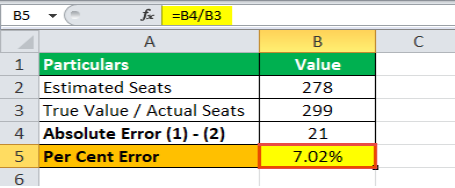Tech and Science Ways to find the percent error in your measurementsWays to find the percent error in your measurements

Are you really concerned about the accuracy of your experiments? In other words you may want to know the percentage difference between the actual and final results. Complete accuracy in the results may not be possible, because of some unintentional human errors.

We cannot completely eliminate the chances of error, but we can devise ways to reduce these errors. For example, in some chemistry experiments, we calculate the actual yield of the chemical reactions and then compare it with the “theoretical yield”.

When we know the percentage error, we can try and standardize our experiment, i.e. careful adding of the solvents, avoiding spillage etc. In this article, we will emphasize on ways to find out the percentage of error in our routine measurements.

Now, let’s get started, here are a few of the best possible methods of calculating percent yield:

1) Online smart calculators:

One of the easiest to use method is to get help from an online tool. You may find a bunch of online tools that are smart enough to compute any problem you feed it with.

One such tool is a “percent error calculator”, this easy to use gizmo is well equipped in finding the error percentage, given the true and observed value. All you need to do is enter the required values in the given fields and press calculate.

No need to work out with formulas and calculations, here just leave the math work on this online tool and relax. The advanced digital technology has really made our lives much easier.

2) Percent error Formula:

Now, let’s get to basics and calculate the percentage of error in our findings, using the formula. Before moving towards formula, know some of the terms. True value means the expected value that should have been acquired at the end of the results, if our findings were to be error free.

On the other hand, the observed value is the value obtained having some errors, most often human error.

Percent error = True value – observed value/ True value * 100

Assume, you are conducting a physics experiment, regarding the speed of sound.

Here, True value of speed of sound is 343 meter per second in air at 20◦c.

The value observed after the experiment was 332 m/s.

Percent error = 343 – 332 / 343 * 100 = 11/343* 100 = 3.2%

3) Use excel:

You can take help from excel, just enter the data, true and observed values in separate cells. Then, using the same formula mentioned above, you can easily calculate the percent error with speed and precision without doing any calculation on your own.